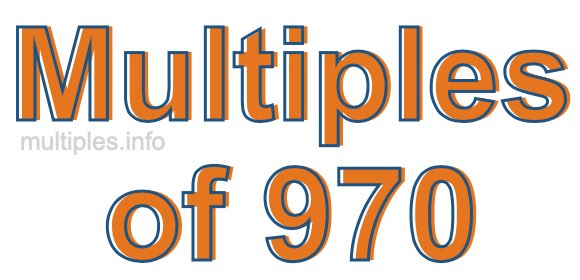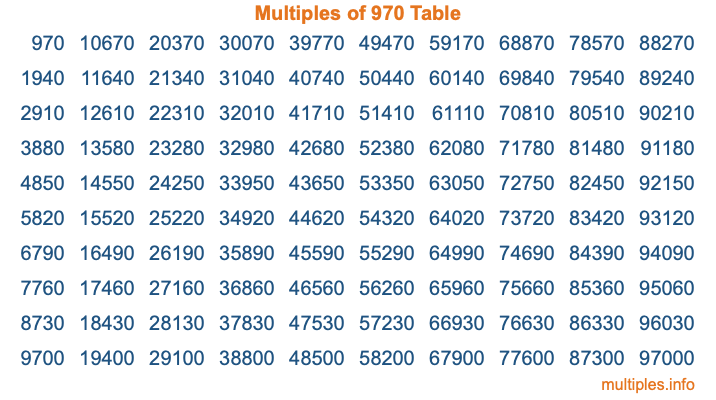Multiples of 970Welcome to the Multiples of 970 page. Here we will first teach you everything you will ever need to know about the multiples of 970, and then give you a study guide summary of everything we taught you to make sure you remember it all. Use this page to look up facts and learn information about the multiples of 970. This page will make you a multiples of nine hundred seventy expert!

Definition of Multiples of 970
Multiples of 970 are all the numbers that when divided by 970 equal an integer. Each of the multiples of 970 are called a multiple. A multiple of 970 is created by multiplying 970 by an integer.

Therefore, to create a list of multiples of 970, you start with 1 multiplied by 970, then 2 multiplied by 970, then 3 multiplied by 970, and so on for as long as you want. Thus, the list of the first five multiples of 970 is 970, 1940, 2910, 3880, and 4850. To see a larger list of multiples of 970, see the printable image of Multiples of 970 further down on this page. We also have a category where you can choose any nth multiple of 970.

Multiples of 970 Checker
The Multiples of 970 Checker below checks to see if any number of your choice is a multiple of 970. In other words, it checks to see if there is any number (integer) that when multiplied by 970 will equal your number. To do that, we divide your number by 970. If the the quotient is an integer, then your number is a multiple of 970.

Is  a multiple of 970?

Least Common Multiple of 970 and ...
A Least Common Multiple (LCM) is the lowest multiple that two or more numbers have in common. This is also called the smallest common multiple or lowest common multiple and is useful to know when you are adding our subtracting fractions. Enter one or more numbers below (970 is already entered) to find the LCM.

Check out our LCM Calculator if you need more details about the Least Common Multiple or if you need the LCM for different numbers for adding and subtraction fractions.

nth Multiple of 970
As we stated above, 970 is the first multiple of 970, 1940 is the second multiple of 970, 2910 is the third multiple of 970, and so on. Enter a number below to find the nth multiple of 970.

th multiple of 970

Multiples of 970 vs Factors of 970
970 is a multiple of 970 and a factor of 970, but that is where the similarities end. All postive multiples of 970 are 970 or greater than 970. All positive factors of 970 are 970 or less than 970.

Below is the beginning list of multiples of 970 and the factors of 970 so you can compare:

Multiples of 970: 970, 1940, 2910, 3880, 4850, etc.

Factors of 970: 1, 2, 5, 10, 97, 194, 485, 970

As you can see, the multiples of 970 are all the numbers that you can divide by 970 to get a whole number. The factors of 970, on the other hand, are all the whole numbers that you can multiply by another whole number to get 970.

It's also interesting to note that if a number (x) is a factor of 970, then 970 will also be a multiple of that number (x).

Multiples of 970 vs Divisors of 970
The divisors of 970 are all the integers that 970 can be divided by evenly. Below is a list of the divisors of 970.

Divisors of 970: 1, 2, 5, 10, 97, 194, 485, 970

The interesting thing to note here is that if you take any multiple of 970 and divide it by a divisor of 970, you will see that the quotient is an integer.

Multiples of 970 Table
Below is an image of the first 100 multiples of 970 in a table. The table is in chronological order, column by column. The first column has the first ten multiples of 970, the second column has the next ten multiples of 970, and so on.The Multiples of 970 Table is also referred to as the 970 Times Table or Times Table of 970. You are welcome to print out our table for your studies.

Negative Multiples of 970
Although not often discussed or needed in math, it is worth mentioning that you can make a list of negative multiples of 970 by multiplying 970 by -1, then by -2, then by -3, and so on, to get the following list of negative multiples of 970:

-970, -1940, -2910, -3880, -4850, etc.

Multiples of 970 Summary
Below is a summary of important Multiples of 970 facts that we have discussed on this page. To retain the knowledge on this page, we recommend that you read through the summary and explain to yourself or a study partner why they hold true.

There are an infinite number of multiples of 970.

A multiple of 970 divided by 970 will equal a whole number.

970 divided by a factor of 970 equals a divisor of 970.

The nth multiple of 970 is n times 970.

The largest factor of 970 is equal to the first positive multiple of 970.

970 is a multiple of every factor of 970.

970 is a multiple of 970.

A multiple of 970 divided by a divisor of 970 equals an integer.

970 divided by a divisor of 970 equals a factor of 970.

Any integer times 970 will equal a multiple of 970.

Multiples of a Number
Here you can get the multiples of another number, all with the same attention to detail as we did for multiples of 970 on this page.

Multiples of
Multiples of 971
Did you find our page about multiples of nine hundred seventy educational? Do you want more knowledge? Check out the multiples of the next number on our list!